NEET  >  NCERT Solutions: Electromagnetic Waves

# NCERT Solutions: Electromagnetic Waves - Notes | Study Physics Class 12 - NEET

 1 Crore+ students have signed up on EduRev. Have you?

NCERT QUESTIONS

(Electromagnetic Waves)

Ques 8.1: Figure 8.6 shows a capacitor made of two circular plates each of radius 12 cm, and separated by 5.0 cm. The capacitor is being charged by an external source (not shown in the figure). The charging current is constant and equal to 0.15 A.

(a) Calculate the capacitance and the rate of charge of potential difference between the plates.

(b) Obtain the displacement current across the plates.

(c) Is Kirchhoff’s first rule (junction rule) valid at each plate of the capacitor? Explain.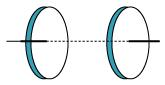Ans:  Radius of each circular plate, r = 12 cm = 0.12 m

Distance between the plates, d = 5 cm = 0.05 m

Charging current, I = 0.15 A

Permittivity of free space,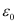= 8.85 × 10−12 C2 N−1 m−2

(a) Capacitance between the two plates is given by the relation,

C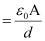Where,

A = Area of each plate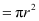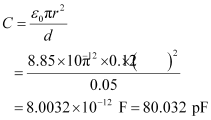Charge on each plate, qCV

Where,

V = Potential difference across the plates

Differentiation on both sides with respect to time (t) gives: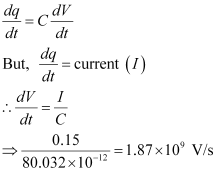Therefore, the change in potential difference between the plates is 1.87 ×109 V/s.

(b) The displacement current across the plates is the same as the conduction current. Hence, the displacement current, id is 0.15 A.

(c) Yes

Kirchhoff’s first rule is valid at each plate of the capacitor provided that we take the sum of conduction and displacement for current.

Ques 8.2:  A parallel plate capacitor (Fig. 8.7) made of circular plates each of radius R = 6.0 cm has a capacitance C = 100 pF. The capacitor is connected to a 230 V ac supply with a (angular) frequency of 300 rad s−1.

(a) What is the rms value of the conduction current?

(b) Is the conduction current equal to the displacement current?

(c) Determine the amplitude of B at a point 3.0 cm from the axis between the plates.Ans:  Radius of each circular plate, R = 6.0 cm = 0.06 m

Capacitance of a parallel plate capacitor, C = 100 pF = 100 × 10−12 F

Supply voltage, V = 230 V

Angular frequency, ω = 300 rad s−1

(a) Rms value of conduction current, I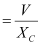Where,

XC = Capacitive reactance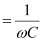∴ IV × ωC

= 230 × 300 × 100 × 10−12

= 6.9 × 10−6 A

= 6.9 μA

Hence, the rms value of conduction current is 6.9 μA.

(b) Yes, conduction current is equal to displacement current.

(c) Magnetic field is given as:

B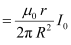Where,

μ0 = Free space permeability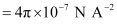I0 = Maximum value of current =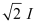r = Distance between the plates from the axis = 3.0 cm = 0.03 m

B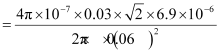= 1.63 × 10−11 T

Hence, the magnetic field at that point is 1.63 × 10−11 T.

Ques 8.3: What physical quantity is the same for X-rays of wavelength 10−10 m, red light of wavelength 6800 Å and radiowaves of wavelength 500 m?

Ans:  The speed of light (3 × 108 m/s) in a vacuum is the same for all wavelengths. It is independent of the wavelength in the vacuum.

Ques 8.4: A plane electromagnetic wave travels in vacuum along z-direction. What can you say about the directions of its electric and magnetic field vectors? If the frequency of the wave is 30 MHz, what is its wavelength?

Ans:  The electromagnetic wave travels in a vacuum along the z-direction. The electric field (E) and the magnetic field (H) are in the x-y plane. They are mutually perpendicular.

Frequency of the wave, ν = 30 MHz = 30 × 106 s−1

Speed of light in a vacuum, c = 3 × 108 m/s

Wavelength of a wave is given as: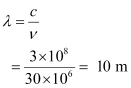Ques 8.5: A radio can tune in to any station in the 7.5 MHz to 12 MHz band. What is the corresponding wavelength band?

Ans:  A radio can tune to minimum frequency, ν1 = 7.5 MHz= 7.5 × 106 Hz

Maximum frequency, ν2 = 12 MHz = 12 × 106 Hz

Speed of light, c = 3 × 108 m/s

Corresponding wavelength for ν1 can be calculated as: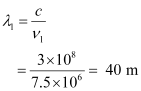Corresponding wavelength for ν2 can be calculated as: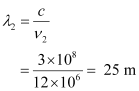Thus, the wavelength band of the radio is 40 m to 25 m.

Ques 8.6: A charged particle oscillates about its mean equilibrium position with a frequency of 109 Hz. What is the frequency of the electromagnetic waves produced by the oscillator?

Ans:  The frequency of an electromagnetic wave produced by the oscillator is the same as that of a charged particle oscillating about its mean position i.e., 109 Hz.

Ques 8.7: The amplitude of the magnetic field part of a harmonic electromagnetic wave in vacuum is B0 = 510 nT. What is the amplitude of the electric field part of the wave?

Ans:  Amplitude of magnetic field of an electromagnetic wave in a vacuum,

B0 = 510 nT = 510 × 10−9 T

Speed of light in a vacuum, c = 3 × 108 m/s

Amplitude of electric field of the electromagnetic wave is given by the relation,

EcB0

= 3 × 108 × 510 × 10−9 = 153 N/C

Therefore, the electric field part of the wave is 153 N/C.

Ques 8.8: Suppose that the electric field amplitude of an electromagnetic wave is E0 = 120 N/C and that its frequency is ν = 50.0 MHz. (a) Determine, B0, ω, k, and λ. (b) Find expressions for E and B.

Ans:  Electric field amplitude, E0 = 120 N/C

Frequency of source, ν = 50.0 MHz = 50 × 106 Hz

Speed of light, c = 3 × 108 m/s

(a) Magnitude of magnetic field strength is given as: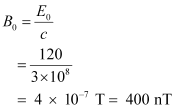Angular frequency of source is given as:

ω = 2πν

= 2π × 50 × 106

Propagation constant is given as: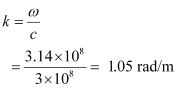Wavelength of wave is given as: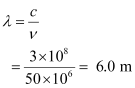(b) Suppose the wave is propagating in the positive x direction. Then, the electric field vector will be in the positive y direction and the magnetic field vector will be in the positive z direction. This is because all three vectors are mutually perpendicular.

Equation of electric field vector is given as: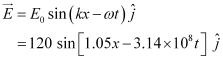And, magnetic field vector is given as: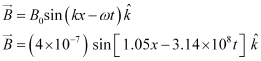Ques 8.9: The terminology of different parts of the electromagnetic spectrum is given in the text. Use the formula E = (for energy of a quantum of radiation: photon) and obtain the photon energy in units of eV for different parts of the electromagnetic spectrum. In what way are the different scales of photon energies that you obtain related to the sources of electromagnetic radiation?

Ans:  Energy of a photon is given as: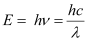Where,

h = Planck’s constant = 6.6 × 10−34 Js

c = Speed of light = 3 × 108 m/s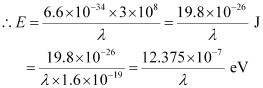The given table lists the photon energies for different parts of an electromagnetic spectrum for differentλ.

 λ(m) 103 1 10-2 10-6 10-8 10-10 10-12 E(eV) 12.375 x10-10 12.375 x10-7 12.375 x10-6 12.375 x10-1 12.375 x101 12,375 x102 12.375 x106

The photon energies for the different parts of the spectrum of a source indicate the spacing of the relevant energy levels of the source.

Ques 8.10: In a plane electromagnetic wave, the electric field oscillates sinusoidally at a frequency of 2.0 × 1010 Hz and amplitude 48 V m−1.

(a) What is the wavelength of the wave?

(b) What is the amplitude of the oscillating magnetic field?

(c) Show that the average energy density of the E field equals the average energy density of the B field. [c = 3 × 108 m s−1.]

Ans:  Frequency of the electromagnetic wave, ν = 2.0 × 1010 Hz

Electric field amplitude, E0 = 48 V m−1

Speed of light, c = 3 × 108 m/s

(a) Wavelength of a wave is given as: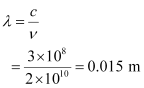(b) Magnetic field strength is given as: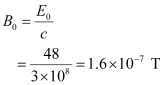(c) Energy density of the electric field is given as: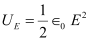And, energy density of the magnetic field is given as: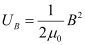Where,

0 = Permittivity of free space

μ0 = Permeability of free space

We have the relation connecting E and B as:

EcB … (1)

Where,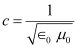… (2)

Putting equation (2) in equation (1), we get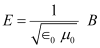Squaring both sides, we get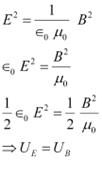Ques 8.11: Suppose that the electric field part of an electromagnetic wave in vacuum is E = {(3.1 N/C) cos [(1.8 rad/m) y  (5.4 × 106 rad/s)t]}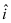.

(a) What is the direction of propagation?

(b) What is the wavelength λ?

(c) What is the frequency ν?

(d) What is the amplitude of the magnetic field part of the wave?

(e) Write an expression for the magnetic field part of the wave.

Ans:  (a) From the given electric field vector, it can be inferred that the electric field is directed along the negative x direction. Hence, the direction of motion is along the negative y direction i.e.,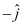.

(b) It is given that,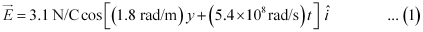The general equation for the electric field vector in the positive x direction can be written as: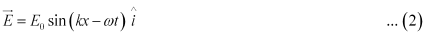On comparing equations (1) and (2), we get

Electric field amplitude, E0 = 3.1 N/C

Angular frequency, ω = 5.4 × 108 rad/s

Wave number, k = 1.8 rad/m

Wavelength,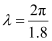= 3.490 m

(c) Frequency of wave is given as: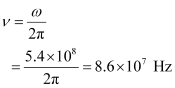(d) Magnetic field strength is given as: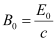Where,

c = Speed of light = 3 × 108 m/s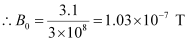(e) On observing the given vector field, it can be observed that the magnetic field vector is directed along the negative z direction. Hence, the general equation for the magnetic field vector is written as: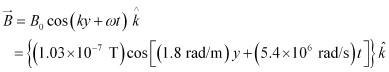Ques 8.12: About 5% of the power of a 100 W light bulb is converted to visible radiation. What is the average intensity of visible radiation

(a) at a distance of 1 m from the bulb?

(b) at a distance of 10 m?

Assume that the radiation is emitted isotropically and neglect reflection.

Ans:  Power rating of bulb, P = 100 W

It is given that about 5% of its power is converted into visible radiation.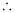Power of visible radiation,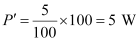Hence, the power of visible radiation is 5W.

(a) Distance of a point from the bulb, d = 1 m

Hence, intensity of radiation at that point is given as: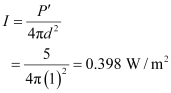(b) Distance of a point from the bulb, d1 = 10 m

Hence, intensity of radiation at that point is given as: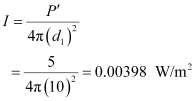Ques 8.13: Use the formula λm T= 0.29 cm K to obtain the characteristic temperature ranges for different parts of the electromagnetic spectrum. What do the numbers that you obtain tell you?

Ans:  A body at a particular temperature produces a continuous spectrum of wavelengths. In case of a black body, the wavelength corresponding to maximum intensity of radiation is given according to Planck’s law. It can be given by the relation,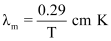Where,

λm = maximum wavelength

T = temperature

Thus, the temperature for different wavelengths can be obtained as:

For λm = 10−4 cm;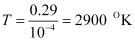For λm = 5 ×10−5 cm;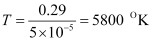For λm = 10−6 cm;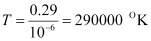and so on.

The numbers obtained tell us that temperature ranges are required for obtaining radiations in different parts of an electromagnetic spectrum. As the wavelength decreases, the corresponding temperature increases.

Ques 8.14: Given below are some famous numbers associated with electromagnetic radiations in different contexts in physics. State the part of the electromagnetic spectrum to which each belongs.

(a) 21 cm (wavelength emitted by atomic hydrogen in interstellar space).

(b) 1057 MHz (frequency of radiation arising from two close energy levels in hydrogen; known as Lamb shift).

(c) 2.7 K [temperature associated with the isotropic radiation filling all space-thought to be a relic of the ‘big-bang’ origin of the universe].

(d) 5890 Å - 5896 Å [double lines of sodium]

(e) 14.4 keV [energy of a particular transition in 57Fe nucleus associated with a famous high resolution spectroscopic method

(Mössbauer spectroscopy)].

Ans:  (a) Radio waves; it belongs to the short wavelength end of the electromagnetic spectrum.

(b) Radio waves; it belongs to the short wavelength end.

(c) Temperature, T = 2.7 °K

λm is given by Planck’s law as: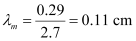This wavelength corresponds to microwaves.

(d) This is the yellow light of the visible spectrum.

(e) Transition energy is given by the relation,

E

Where,

h = Planck’s constant = 6.6 × 10−34 Js

Energy, E = 14.4 K eV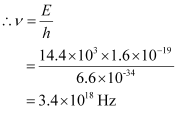This corresponds to X-rays.

Ques 8.15: Answer the following questions:

(b) It is necessary to use satellites for long distance TV transmission. Why?

(c) Optical and radio telescopes are built on the ground but X-ray astronomy is possible only from satellites orbiting the earth. Why?

(d) The small ozone layer on top of the stratosphere is crucial for human survival. Why?

(e) If the earth did not have an atmosphere, would its average surface temperature be higher or lower than what it is now?

(f) Some scientists have predicted that a global nuclear war on the earth would be followed by a severe ‘nuclear winter’ with a devastating effect on life on earth. What might be the basis of this prediction?

Ans:  (a) Long distance radio broadcasts use shortwave bands because only these bands can be refracted by the ionosphere.

(b) It is necessary to use satellites for long distance TV transmissions because television signals are of high frequencies and high energies. Thus, these signals are not reflected by the ionosphere. Hence, satellites are helpful in reflecting TV signals. Also, they help in long distance TV transmissions.

(c) With reference to X-ray astronomy, X-rays are absorbed by the atmosphere. However, visible and radio waves can penetrate it. Hence, optical and radio telescopes are built on the ground, while X-ray astronomy is possible only with the help of satellites orbiting the Earth.

(d) The small ozone layer on the top of the atmosphere is crucial for human survival because it absorbs harmful ultraviolet radiations present in sunlight and prevents it from reaching the Earth’s surface.

(e) In the absence of an atmosphere, there would be no greenhouse effect on the surface of the Earth. As a result, the temperature of the Earth would decrease rapidly, making it chilly and difficult for human survival.

(f) A global nuclear war on the surface of the Earth would have disastrous consequences. Post-nuclear war, the Earth will experience severe winter as the war will produce clouds of smoke that would cover maximum parts of the sky, thereby preventing solar light form reaching the atmosphere. Also, it will lead to the depletion of the ozone layer.

The document NCERT Solutions: Electromagnetic Waves - Notes | Study Physics Class 12 - NEET is a part of the NEET Course Physics Class 12.
All you need of NEET at this link: NEET

## Physics Class 12

157 videos|452 docs|213 tests
 Use Code STAYHOME200 and get INR 200 additional OFF

## Physics Class 12

157 videos|452 docs|213 tests

### How to Prepare for NEET

Read our guide to prepare for NEET which is created by Toppers & the best Teachers

Track your progress, build streaks, highlight & save important lessons and more!

,

,

,

,

,

,

,

,

,

,

,

,

,

,

,

,

,

,

,

,

,

;